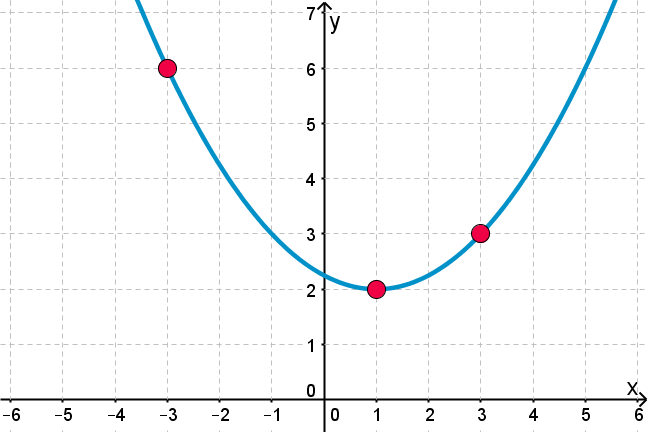## Want to keep learning?

This content is taken from the UNSW Sydney's online course, Maths for Humans: Linear, Quadratic & Inverse Relations. Join the course to learn more.
2.27

## UNSW SydneyParabola through three points

# A quadratic function through three points

Another application of quadratic functions is to curve fitting, also called the theory of splines. Since a parabola $$\normalsize{y=ax^2+bx+c}$$ is specified by three numbers, it is reasonable to suppose that we could fit a parabola to three points in the plane. This is indeed the case, and it is a useful idea.

In this step we see how to algebraically fit a parabola to three points in the Cartesian plane. This involves recalling, or learning, how to solve three equations in three unknowns. This is a useful skill on its own right.

## The unique circle through three non-collinear points

A line is determined by two points. A circle, on the other hand, is determined by three points —as long as these points are not collinear (all three points cannot lie on the same line). The construction of a circle which passes through three points is a standard exercise in Euclidean geometry: we construct the perpendicular bisectors of the line segments determined by these three points, and then these three lines meet at the circumcenter of the triangle $$\normalsize{ABC}$$, namely the centre of the unique circle which passes through all three points.

Here is a GeoGebra image of this construction:What about a parabola? Well, if we restrict ourselves to parabolas given by quadratic equations, that is curves which the form $$\normalsize{y=ax^2+bx+c}$$, then since there are three unknown quantities, we can expect that we can fit such a curve to three distinct points. Let’s see how this works in practice.

## Fitting a parabola to three points

Suppose we want to find a parabola with equation $$\normalsize{y=ax^2+bx+c}$$ which passes through the points $$\normalsize{[0,1], [1,5]}$$ and $$\normalsize{[2,3]}$$. Substituting each of the three points into the equation, we get

$\Large{1=c}$ $\Large{5=a+b+c}$ $\Large{3=4a+2b+c}$

This is three equations in three unknowns. This is more complicated, but in this particular case, things are simpler since the first equation already tells us that $$\normalsize{c=1}$$, so the other two equations become $$\normalsize{a+b=4}$$ and $$\normalsize{2a+b=1}$$.

Solving these equations, yields $$\normalsize{a=-3}$$, $$\normalsize{b=7}$$ and also $$\normalsize{c=1}$$, so the required parabola is $$\normalsize{y=-3x^2+7x+1}$$. Here is the graph:## Solving three linear equations in three unknowns

Since two linear equations represent two lines in the plane, their common solution corresponds to the geometric meet of the two lines. For three linear equations in three unknowns, the situation actually corresponds to the common intersection point of three planes in three-dimensional space!

Fortunately the ancient Chinese were able to develop a general technique for solving such systems of equations. Here we just try to find a simple practical method.

Suppose we want to find the equation of the quadratic function $$\normalsize{y=ax^2+bx+c}$$ which passes through the points $$\normalsize{[1,3], [2,-1]}$$ and $$\normalsize{[4,1]}$$. It means we have three equations, one for each of the points – since we know the points given must satisfy the unknown equation. The three equations are

$\Large{3=a+b+c} \label{b1p} \tag {1}$ $\Large{-1=4a+2b+c} \label{b2p} \tag {2}$ $\Large{1=16a+4b+c}. \label{b3p} \tag 3$

What is the strategy? It is simple: we try to eliminate one of the variables, leaving us with two equations in two unknowns. This we know how to solve.

To get two equations in two variables, let’s eliminate $$\normalsize{c}$$ from the first two equations. We do that by subtracting one from the other, say subtract the first from the second:

$\Large{-4=3a+b} \label{b4p} \tag{4}$

Please make sure you understand how we got that. Now we do the same with the last two equations: take the third equation and subtract the second:

$\Large{2=12a+2b}$

We can make that a bit simpler by dividing all the coefficients by $$\normalsize{2}$$ to get

$\Large{1=6a+b}. \label{b5p} \tag {5}$

Now we treat $$\normalsize{(\ref{b5p})}$$ and $$\normalsize{(\ref{b4p})}$$ as new equations, and use them to find $$\normalsize{a}$$ and $$\normalsize{b}$$.

If we take $$\normalsize{(\ref{b5p})}$$–$$\normalsize{(\ref{b4p})}$$ we get $$\normalsize{5=3a}$$, so that $$\normalsize{a=5/3}$$, and then plugging back into either $$\normalsize{(\ref{b3p})}$$ or $$\normalsize{(\ref{b4p})}$$ gives $$\normalsize{b=-9}$$. Then putting both of these back into say $$\normalsize{(\ref{b1p})}$$ gives $$\normalsize{3=5/3-9+c}$$ so that $$\normalsize{c=31/3}$$.

The parabola is therefore $$\normalsize{y=5/3x^2-9x+31/3}$$. Here is its graph:Q1 (M): Find the equation of the quadratic function $$\normalsize{y=ax^2+bx+c}$$ which passes through the points $$\normalsize{[-1,9], [2,3]}$$ and $$\normalsize{[5,15]}$$.

Q2 (C): Find a quadratic relation of the form $$\normalsize{x=ay^2 + by +c}$$ which passes through the points $$\normalsize{[0,1], [1,5]}$$ and $$\normalsize{[2,3]}$$.

A1. The answer is the parabolic function $$\normalsize{y=x^2-3x+5}$$.
A2. The answer is the curve $$x=- \frac{1}{8}\left(3y^2-20y+17\right)$$. Note this is not a function.